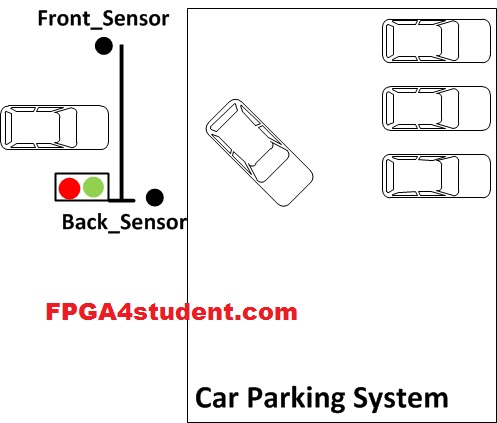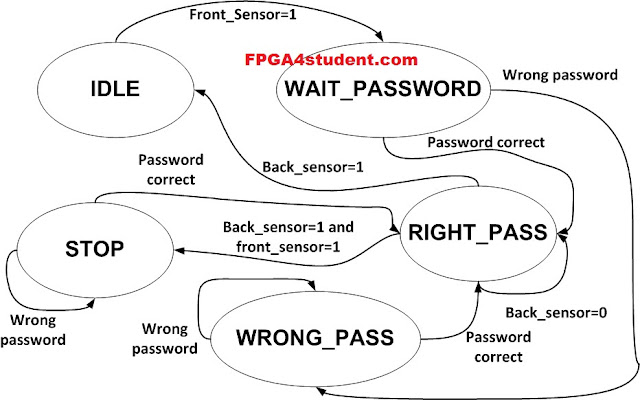# Car Parking System in VHDL

## This VHDL project presents a car parking system in VHDL using Finite State Machine (FSM). VHDL code and testbench for the car parking system are fully provided.

#### The VHDL car parking system is shown in the following figure. There is a front sensor to detect vehicles going to the gate of the car parking system. Another back sensor is to detect if the coming vehicle passed the gate and getting into the car park.#### The car parking system in VHDL operates under the control of a Finite State Machine (FSM) as follows:Initially, the FSM is in IDLE state. If there is a vehicle coming detected by the front sensor, FSM is switched to WAIT_PASSWORD state for 4 cycles. The car will input the password in this state; if the password is correct, the gate is opened to let the car get in the car park and FSM turns to RIGHT_PASS state; a Green LED will be blinking. Otherwise, FSM turns to WRONG_PASS state; a Red LED will be blinking and it requires the car to enter the password again until the password is correct. When the current car gets into the car park detected by the back sensor and there is the next car coming, the FSM is switched to STOP state and the Red LED will be blinking so that the next car will be noticed to stop and enter the password. After the car passes the gate and gets into the car park, the FSM returns to IDLE state.

### VHDL code for the car parking system using FSM:

```-- fpga4student.com FPGA projects, Verilog projects, VHDL projects
-- VHDL project: VHDL code for car parking system
library IEEE;
use IEEE.STD_LOGIC_1164.ALL;
use IEEE.std_logic_unsigned.all;
entity Car_Parking_System_VHDL is
port
(
clk,reset_n: in std_logic; -- clock and reset of the car parking system
front_sensor, back_sensor: in std_logic; -- two sensor in front and behind the gate of the car parking system
GREEN_LED,RED_LED: out std_logic; -- signaling LEDs
HEX_1, HEX_2: out std_logic_vector(6 downto 0) -- 7-segment Display
);
end Car_Parking_System_VHDL;

architecture Behavioral of Car_Parking_System_VHDL is
-- FSM States
signal current_state,next_state: FSM_States;
signal counter_wait: std_logic_vector(31 downto 0);
signal red_tmp, green_tmp: std_logic;

begin
-- Sequential circuits
process(clk,reset_n)
begin
if(reset_n='0') then
current_state <= IDLE;
elsif(rising_edge(clk)) then
current_state <= next_state;
end if;
end process;
-- combinational logic
-- fpga4student.com FPGA projects, Verilog projects, VHDL projects
begin
case current_state is
when IDLE =>
if(front_sensor = '1') then -- if the front sensor is on,
-- there is a car going to the gate
else
next_state <= IDLE;
end if;
if(counter_wait <= x"00000003") then
else -- check password after 4 clock cycles
next_state <= RIGHT_PASS; -- if password is correct, let them in
else
next_state <= WRONG_PASS; -- if not, tell them wrong pass by blinking Green LED
-- let them input the password again
end if;
end if;
when WRONG_PASS =>
next_state <= RIGHT_PASS;-- if password is correct, let them in
else
next_state <= WRONG_PASS;-- if not, they cannot get in until the password is right
end if;
when RIGHT_PASS =>
if(front_sensor='1' and back_sensor = '1') then
next_state <= STOP;
-- if the gate is opening for the current car, and the next car come,
-- STOP the next car and require password
-- the current car going into the car park
elsif(back_sensor= '1') then
-- if the current car passed the gate an going into the car park
-- and there is no next car, go to IDLE
next_state <= IDLE;
else
next_state <= RIGHT_PASS;
end if;
when STOP =>
-- check password of the next car
-- if the pass is correct, let them in
next_state <= RIGHT_PASS;
else
next_state <= STOP;
end if;
when others => next_state <= IDLE;
end case;
end process;
process(clk,reset_n)
begin
if(reset_n='0') then
counter_wait <= (others => '0');
elsif(rising_edge(clk))then
counter_wait <= counter_wait + x"00000001";
else
counter_wait <= (others => '0');
end if;
end if;
end process;
-- output
process(clk) -- change this clock to change the LED blinking period
begin
if(rising_edge(clk)) then
case(current_state) is
when IDLE =>
green_tmp <= '0';
red_tmp <= '0';
HEX_1 <= "1111111"; -- off
HEX_2 <= "1111111"; -- off
green_tmp <= '0';
red_tmp <= '1';
-- RED LED turn on and Display 7-segment LED as EN to let the car know they need to input password
HEX_1 <= "0000110"; -- E
HEX_2 <= "0101011"; -- n
when WRONG_PASS =>
red_tmp <= not red_tmp;
HEX_1 <= "0000110"; -- E
HEX_2 <= "0000110"; -- E
when RIGHT_PASS =>
green_tmp <= not green_tmp;
HEX_1 <= "0000010"; -- 6
HEX_2 <= "1000000"; -- 0
when STOP =>
green_tmp <= '0';
red_tmp <= not red_tmp; -- Stop the next car and RED LED blinking
HEX_1 <= "0010010"; -- 5
HEX_2 <= "0001100"; -- P
when others =>
green_tmp <= '0';
red_tmp <= '0';
HEX_1 <= "1111111"; -- off
HEX_2 <= "1111111"; -- off
end case;
end if;
end process;
RED_LED <= red_tmp  ;
GREEN_LED <= green_tmp;

end Behavioral;```

### VHDL Testbench code for the car parking system using FSM:

```-- fpga4student.com FPGA projects, Verilog projects, VHDL projects
-- VHDL project: VHDL code for car parking system
-- Testbench code for car parking system in VHDL
LIBRARY ieee;
USE ieee.std_logic_1164.ALL;

ENTITY tb_car_parking_system_VHDL IS
END tb_car_parking_system_VHDL;

ARCHITECTURE behavior OF tb_car_parking_system_VHDL IS

-- Component Declaration for the car parking system in VHDL

COMPONENT Car_Parking_System_VHDL
PORT(
clk : IN  std_logic;
reset_n : IN  std_logic;
front_sensor : IN  std_logic;
back_sensor : IN  std_logic;
password_1 : IN  std_logic_vector(1 downto 0);
password_2 : IN  std_logic_vector(1 downto 0);
GREEN_LED : OUT  std_logic;
RED_LED : OUT  std_logic;
HEX_1 : OUT  std_logic_vector(6 downto 0);
HEX_2 : OUT  std_logic_vector(6 downto 0)
);
END COMPONENT;

--Inputs
signal clk : std_logic := '0';
signal reset_n : std_logic := '0';
signal front_sensor : std_logic := '0';
signal back_sensor : std_logic := '0';
signal password_1 : std_logic_vector(1 downto 0) := (others => '0');
signal password_2 : std_logic_vector(1 downto 0) := (others => '0');

--Outputs
signal GREEN_LED : std_logic;
signal RED_LED : std_logic;
signal HEX_1 : std_logic_vector(6 downto 0);
signal HEX_2 : std_logic_vector(6 downto 0);

-- Clock period definitions
constant clk_period : time := 10 ns;

BEGIN

-- Instantiate the car parking system in VHDL
Car_park_system: Car_Parking_System_VHDL PORT MAP (
clk => clk,
reset_n => reset_n,
front_sensor => front_sensor,
back_sensor => back_sensor,
GREEN_LED => GREEN_LED,
RED_LED => RED_LED,
HEX_1 => HEX_1,
HEX_2 => HEX_2
);

-- Clock process definitions
clk_process :process
begin
clk <= '0';
wait for clk_period/2;
clk <= '1';
wait for clk_period/2;
end process;
-- Stimulus process
stim_proc: process
begin
reset_n <= '0';
front_sensor <= '0';
back_sensor <= '0';
wait for clk_period*10;
reset_n <= '1';
wait for clk_period*10;
front_sensor <= '1';
wait for clk_period*10;
wait until HEX_1 = "0000010";
back_sensor <= '1';
wait until HEX_1 = "0010010"; -- stop the next car and require password
front_sensor <= '0';
wait until HEX_1 = "0000010";
back_sensor <= '1';
wait until HEX_1 = "1111111";
back_sensor <= '0';

wait;
end process;

END;
```

#### Simulation waveform for the car parking system in VHDL:The simulation waveform shows the functional operations of the car parking system in VHDL. You can change the VHDL code to increase the blinking period of the Green LED and Red LED. Also, you can change the period of waiting for password being entered in the FSM VHDL code of the car parking system.
Verilog code for the car parking system: here.

1.Hi, by chance, did you do it in physics?

2.how to map HEX_2 (6 down to 0) in XDC when there are Anode(3 down to 0)?

3.can you just provide the circuit diagram for my mini project